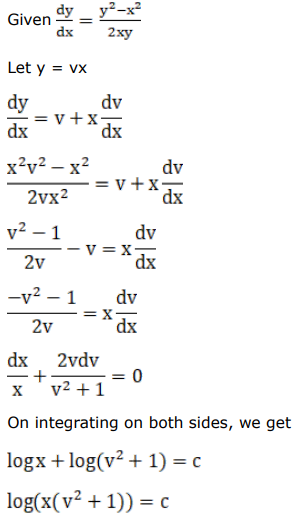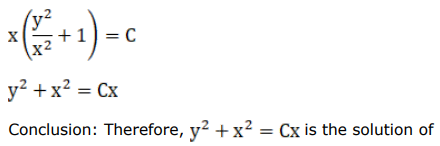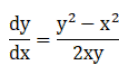# Mark against the correct answer in the following:Question:

Mark $(\sqrt{)}$ against the correct answer in the following:

The general solution of the $D E \frac{d y}{d x}=\frac{y^{2}-x^{2}}{2 x y}$ is

A. $x^{2}-y^{2}=C_{1} x$

B. $x^{2}+y^{2}=C_{1} y$

C. $x^{2}+y^{2}=C_{1} x$

D. None of these

Solution: